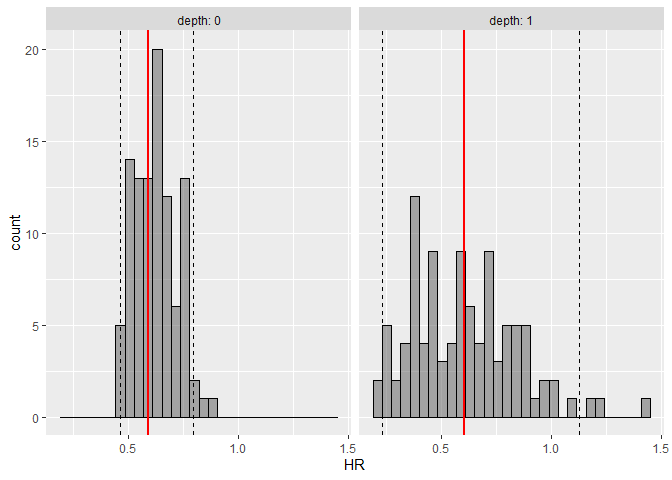Want to share your content on R-bloggers? click here if you have a blog, or here if you don't.

I recently released an R package on CRAN called`survParamSim` for parametric survival simulation, and here want to describe a bit more on details & motivations behind developing this package.

Parametric Survival Simulation with Parameter Uncertainty • survParamSim

The purpose of `survParamSim` is to package the R functions developed by a great scientist, the late Laurent Claret, and streamline the function definition/APIs to make these widely available to the public. Laurent developed these functions for his pioneering works in applying tumor growth inhibition models for clinical outcome predictions of oncology studies, such as the one below. clincancerres.aacrjournals.org

Explaining the theory behind his works is well beyond the scope of this short blog post, but the key technical highlight is that we are using parametric survival models with various covariates for predicting survival profiles and treatment benefits. In applying these workflows in the context of drug development (or maybe in most of other contexts), we want to make a prediction of not only the mean survival profiles but also the uncertainties associated with model predictions.

There are mainly two sources of uncertainty for such survival predictions. The first one is due to the nature of the survival prediction: because survival (or rather failure) is modeled as probabilistic processes, a predicted survival curve for a group will never be identical after multiple simulations. There is another source of uncertainty, which is the uncertainty associated with model parameters, such as coefficients associated with covariates in the model.

The first element is fairly straightforward to evaluate – we can run simulations many times and quantify how variable the survival curves can be by looking at prediction intervals. For example, we expect the prediction interval to generally get narrower if we make a prediction with larger number of patients. However, for the second part, there is not a readily available method for exploration to my (limited) knowledge. `survival::predict.survreg()` has `se.fit` option, but it is not straightforward to carry the output from this option for making predicted survival profiles of a group.

These are the main reasons for putting together this package – to enable the incorporation of above two sources of uncertainty in survival predictions, using parametric models obtained with `survival::survreg()`. For the second element of the uncertainty, we are using parametric bootstrap from variance-covariance matrix of the model parameter estimates.

# Example

The code below is essentially the same as the one on GitHub pages, just showing a simple work flow to give you a sense of what to expect in terms of outputs. Vignette here has a bit more explanations on the workflow.

For this example, we use `colon` dataset in `survival` package, where the treatment benefit of Levamisole+5-FU was compared with control (called as Obs). Various covariates were further explored, such as extent of tumor local spread.

First, you would run `survreg` to fit parametric survival model:

```library(tidyverse)
library(survival)
library(survParamSim)

set.seed(12345)

# ref for dataset https://vincentarelbundock.github.io/Rdatasets/doc/survival/colon.html
colon2 <-
as_tibble(colon) %>%
# recurrence only and not including Lev alone arm
filter(rx != "Lev", etype == 1) %>%
# Same definition as Lin et al, 1994
mutate(rx = factor(rx, levels = c("Obs", "Lev+5FU")),
depth = as.numeric(extent <= 2))```

Here we have two covariates, `node4` and `depth`, in addition to the treatment assignment `rx`.

```fit.colon <- survreg(Surv(time, status) ~ rx + node4 + depth,
data = colon2, dist = "lognormal")```

Next, parametric bootstrap simulation can be run with `surv_param_sim()` function from this package:

```sim <-
surv_param_sim(object = fit.colon, newdata = colon2,
censor.dur = c(1800, 3000),
# Simulating only 100 times to make the example go fast
n.rep = 100)```

The output object merely has raw simulated survival data with 100 repetition (defined by `n.rep` argument). You can find what options you have on this output by simply printing the object.

```sim
#> ---- Simulated survival data with the following model ----
#> survreg(formula = Surv(time, status) ~ rx + node4 + depth, data = colon2,
#>     dist = "lognormal")
#>
#> * Use `extract_sim()` function to extract individual simulated survivals
#> * Use `calc_km_pi()` function to get survival curves and median survival time
#> * Use `calc_hr_pi()` function to get hazard ratio
#>
#> * Settings:
#>     #simulations: 100
#>     #subjects: 619 (without NA in model variables)```

To visualize simulated survival curves with uncertainly, you can use `calc_km_pi()` and `plot_km_pi()` functions sequentially. You can also specify faceting/grouping with `trt` and `group` input arguments.

```km.pi <- calc_km_pi(sim, trt = "rx", group = c("node4", "depth"))

km.pi
#> ---- Simulated and observed (if calculated) survival curves ----
#> * Use `extract_median_surv()` to extract median survival times
#> * Use `extract_km_pi()` to extract prediction intervals of K-M curves
#> * Use `plot_km_pi()` to draw survival curves
#>
#> * Settings:
#>     trt: rx
#>     group: node4
#>     pi.range: 0.95
#>     calc.obs: TRUE

plot_km_pi(km.pi) +
theme(legend.position = "bottom") +
labs(y = "Recurrence free rate") +
expand_limits(y = 0)```

Similarly, you can visualize hazard ratios and prediction intervals with `calc_hr_pi()` and `plot_hr_pi()` functions.

```hr.pi <- calc_hr_pi(sim, trt = "rx", group = c("depth"))

hr.pi
#> ---- Simulated and observed (if calculated) hazard ratio ----
#> * Use `extract_hr_pi()` to extract prediction intervals and observed HR
#> * Use `extract_hr()` to extract individual simulated HRs
#> * Use `plot_hr_pi()` to draw histogram of predicted HR
#>
#> * Settings:
#>     trt: rx
#>          (Lev+5FU as test trt, Obs as control)
#>     group: depth
#>     pi.range: 0.95
#>     calc.obs: TRUE
plot_hr_pi(hr.pi)```Histogram of predicted hazard ratios, prediction intervals (dashed lines), and observed hazard ratios (red line), per depth groups